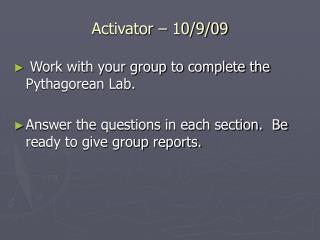DownloadDownload PresentationActivator – 10/9/09

# Activator – 10/9/09

Télécharger la présentation## Activator – 10/9/09

- - - - - - - - - - - - - - - - - - - - - - - - - - - E N D - - - - - - - - - - - - - - - - - - - - - - - - - - -
##### Presentation Transcript

1. Activator – 10/9/09 • Work with your group to complete the Pythagorean Lab. • Answer the questions in each section. Be ready to give group reports.

2. How is the Pythagorean Theorem used? • A right triangle is a triangle with one right angle. • The sides that form the right angle are called the legs. • The hypotenuse is the side opposite the right angle. It is the longest side of the triangle. • The Pythagorean Theorem describes the relationship between the lengths of the legs and the hypotenuse of any right triangle. • Pythagorean Theorem – in a right triangle, the square of the length of the hypotenuse is equal to the sum of the squares of the lengths of the legs. • In-class examples.

3. Summarizer • Solve the following word problems. Hint draw a picture to help you identify what information is given and what is being solved for. • The hypotenuse of a right triangle is 33 centimeters long and one of its legs is 28 centimeters. Find the length of the other leg. • A 10-foot ramp is extended from the top of a truck to the ground to help movers load furniture onto the truck. If the ramp touches the ground at a point 9 feet behind the truck, how high off the ground is the top of the ramp? • The measures of three sides of a triangle are 24 inches, 7 inches, and 25 inches. Determine whether the triangle is a right triangle.